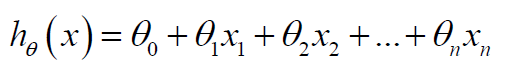### Understanding Multiple/ Multivariate Linear Regression in Machine Learning

In this post we will discuss the following topics

Linear Regression with Multiple Variables (Multivariate/ Multiple Linear Regression)

## Linear Regression with Multiple Variables (Multivariate/ Multiple Linear Regression)

Let's start with example of house price prediction with multiple features (variables).

 Size(feet2) (x1) Number of Bedrooms (x2) Number of Floors (x3) Age of Home (Years) (x4) Price (\$1000) (y) 2010 5 3 30 430 1390 3 2 29 230 1500 2 1 20 315

Here in this example four features Size, number of bedrooms, number of floors and age of home.

Hypothesis:In this example the Hypothesis is the predicted price of the house. Here we have 4 features (variables) and 5 unknown parameters. These parameters can be solved either by normal equation or by the gradient descent method. In general if the total number of features is n, and the total no of training example is m, we will have the Hypothesis as follows

n- total no of features, m-total training examples, xj-input(feature).

Hypothesis:

Parameters: θ0, θ1,..., θn

Cost Function:

### Gradient Descent Method for Multivariate Linear Regression

Procedure:

• Keep changing θ0, θ1,..., θn to reduce J until we hope fully reach at minimum
Here α is Learning Rate. How to choose the learning rate. If α is too small, then the gradient descent can be slow. And if α is too large, gradient descent can overshoot the minimum. It may fail to converge or in worst case it may even diverge.

### Feature Scaling

In the example size :0-2000 feet2 and number of bedrooms:1-5. These two variables cannot be fit on a similar scale. So we need feature scaling for this type of the problems. Feature scaling can be done by dividing the variables by maximum values. In this case the values of variables rage from 0 to 1.

The other way to get the features scaled is Mean Normalization. In mean normalization the mean is subtracted from the variable and then divided by the range of the variable. In this way we will get the variables in the range from -0.5 to 0.5. Mean Normalization is best for the feature scaling.

Also cover feature scaling. Idea of feature scaling is to make all features on a similar scale. The Mean Normalization is one of the best methods of feature scaling. We generally declare convergence if J(θ) decreases by less than 10-3 .

The other Method to solve for Multivariate Linear Regression is Normal Equation. The normal equation is generally recommended to use if no of features is less than 1000 but gradient descent is more useful if number of features is larger value.

### Polynomial Regression

In the Polynomial regression the hypothesis is a polynomial instead a linear equation. the xi's may have powers other than 0 and 1. In our example the price of home may dependent on the square of the size or even no of bedrooms or even it may be square root of the size. With the help of the nature of the curve can be find using the graph of the training data.

### Normal Equation

The Normal equation is other method than gradient descent to find the values of the parameters. In this we have direct formula to calculate the parameters, but the idea behind this is to minimize the cost function. To minimize cost function we partially take derivatives with respect to each parameters and equate them to zero. after solving these equations we will get a normal formula to find parameters.

We have discussed Logistic Regression in Machine Learning in one of our  Machine Learning posts.